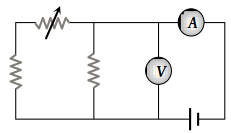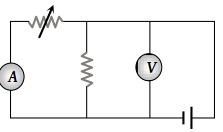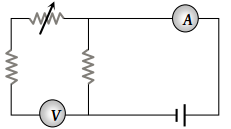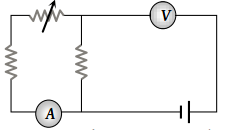A copper wire has a square cross-section, 2.0 mm on a side. It carries a current of 8 A and the density of free electrons is 8 × 1028 m–3. The drift speed of electrons is equal to

(1) 0.156 × 10–3 m.s–1

(2) 0.156 × 10–2 m.s–1

(3) 3.12 × 10–3 m.s–1

(4) 3.12 × 10–2 m.s–1

Concept Questions :-

Current and current density
High Yielding Test Series + Question Bank - NEET 2020

Difficulty Level:

What length of the wire of specific resistance $48×{10}^{-8}\text{\hspace{0.17em}}\Omega \text{\hspace{0.17em}\hspace{0.17em}}m$is needed to make the resistance of 4.2 Ω (diameter of wire = 0.4 mm

(1) 4.1 m

(2) 3.1 m

(3) 2.1 m

(4) 1.1 m

Concept Questions :-

Derivation of Ohm's law
High Yielding Test Series + Question Bank - NEET 2020

Difficulty Level:

A strip of copper and another of germanium are cooled from room temperature to 80 K. The resistance of

(1) Each of these increases

(2) Each of these decreases

(3) Copper strip increases and that of germanium decreases

(4) Copper strip decreases and that of germanium increases

Concept Questions :-

Derivation of Ohm's law
High Yielding Test Series + Question Bank - NEET 2020

Difficulty Level:

The length of a given cylindrical wire is increased by 100%. Due to the consequent decrease in diameter, the change in the resistance of the wire will be :

(1) 300%

(2) 200%

(3) 100%

(4) 50%

Concept Questions :-

Derivation of Ohm's law
High Yielding Test Series + Question Bank - NEET 2020

Difficulty Level:

Express which of the following setups can be used to verify Ohm’s law

 (1)(2)(3)(4)Concept Questions :-

Derivation of Ohm's law
High Yielding Test Series + Question Bank - NEET 2020

Difficulty Level:

In a hydrogen discharge tube, it is observed that through a given cross-section 3.13 × 1015 electrons are moving from right to left and 3.12 × 1015 protons are moving from left to right. What is the electric current in the discharge tube and what is its direction :

(1) 1 mA towards the right

(2) 1 mA towards left

(3) 2 mA towards left

(4) 2 mA towards the right

Concept Questions :-

Current and current density
High Yielding Test Series + Question Bank - NEET 2020

Difficulty Level:

A resistance R is stretched to four times its length. Its new resistance will be

(1) 4 R

(2) 64 R

(3) R/4

(4) 16 R

Concept Questions :-

Derivation of Ohm's law
High Yielding Test Series + Question Bank - NEET 2020

Difficulty Level:

What is the resistance of a carbon resistance which has bands of colours brown, black and brown

(1) 100 Ω

(2) 1000 Ω

(3) 10 Ω

(4) 1 Ω

Concept Questions :-

Colour coding of resistors
High Yielding Test Series + Question Bank - NEET 2020

Difficulty Level:

The electric resistance of a certain wire of iron is R. If its length and radius are both doubled, then :

(1) The resistance will be doubled and the specific resistance will be halved

(2) The resistance will be halved and the specific resistance will remain unchanged

(3) The resistance will be halved and the specific resistance will be doubled

(4) The resistance and the specific resistance, will both remain unchanged

Concept Questions :-

Derivation of Ohm's law
High Yielding Test Series + Question Bank - NEET 2020

Difficulty Level:

The following four wires are made of the same material and are at the same temperature. Which one of them has highest electrical resistance :

(1) Length = 50 cm, diameter = 0.5 mm

(2) Length = 100 cm, diameter = 1 mm

(3) Length = 200 cm, diameter = 2 mm

(4) Length = 300 cm, diameter = 3 mm

Concept Questions :-

Derivation of Ohm's law